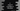# C program to check if a number is Abundant or Excessive## C program to check if a number is Abundant or Excessive number:

In this C program, we will learn how to check if a number is an abundant number or not. We will write a program that will take a number as the input, verify if it is an abundant number or not and print a message to the user.

We will learn what is an abundant number and how to write a C program to check if a number is abundant or not in this post.

### What is an abundant number:

A number is called an abundant number or excessive number if the sum of the proper divisors of that number is greater than the number itself.

For example, 12 is an abundant number. Its proper divisors are 1, 2, 3, 4 and 6. The sum is 16. So, 12 is an Abundant number because 16 > 12.

### Algorithm to check if a number is an abundant number or not:

We will use the below algorithm to check if a number is an abundant number or not.

• Take a number as input from the user.
• Initialize a variable as 0 to hold the sum of all proper divisors.
• By using a loop from 1 to number - 1, find if any number is a proper divisor of that number or not.
• If it is a proper divisor, add it to the sum variable.
• Once the loop is completed, compare the sum with the original number. If the sum is greater than the number, it is an abundant number. Else, it is not.

### C program:

Let’s write down the program:

``````#include <stdio.h>

int isAbundant(int no)
{
int sum = 0;

for (int i = 1; i < no; i++)
{
if (no % i == 0)
{
sum += i;
}
}

return sum > no;
}

int main()
{
int no;

printf("Enter a number: ");
scanf("%d", &no);

if (isAbundant(no))
printf("%d is an Abundant number.\n", no);
else
printf("%d is not an Abundant number.\n", no);

return 0;
}``````

Here,

• isAbundant method checks if a number is Abundant or not.

• It initializes a variable sum as 0.
• The for loop runs from i = 1 to i = no - 1.
• On each iteration, it checks if the current value of i is proper divisor of the number or not. We are using the modulo operator to check that. If the result of modulo is 0, it indicates that the number is properly divisible by i.
• If it is a proper divisor, it adds that value to the sum variable.
• It returns 1 if the sum is greater than the number. Else, it returns 0.
• It takes the number as an input and stores that in the variable no.
• It calls isAbundant method to check if that number is an abundant number or not.

### Sample output:

It will print output as like below:

``````Enter a number: 12
12 is an Abundant number.

Enter a number: 13
13 is not an Abundant number.``````

### Method 2: Reduce the steps:

We can reduce the steps in the for loop used to find the perfect divisors. Instead of running this from 1 to number - 1, we can run it from 1 to number/2.

It will be as like below:

``````#include <stdio.h>

int isAbundant(int no)
{
int sum = 0;

for (int i = 1; i <= no / 2; i++)
{
if (no % i == 0)
{
sum += i;
}
}

return sum > no;
}

int main()
{
int no;

printf("Enter a number: ");
scanf("%d", &no);

if (isAbundant(no))
printf("%d is an Abundant number.\n", no);
else
printf("%d is not an Abundant number.\n", no);

return 0;
}``````

If you run this program, it will give similar output.